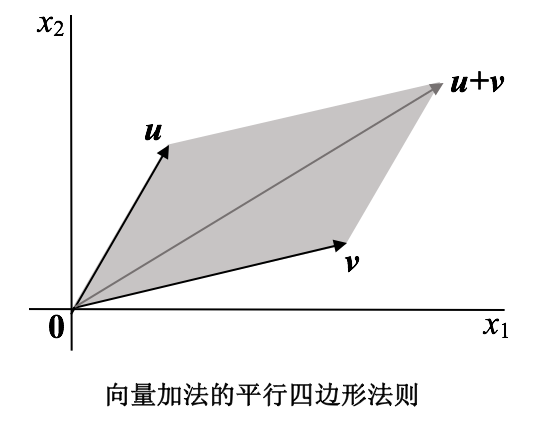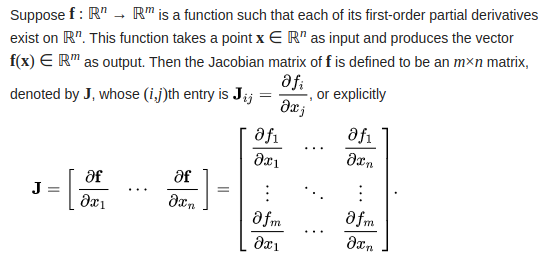• 向量方程的定义和求解 Span{v}\boldsymbol{Span\{v\}}Span{v}与Span{u,v}\boldsymbol{Span\{u,v\}}Span{u,v}的几何解释 R2\mathbb{R}^{2}R2中的向量 仅含一列的矩阵称为&amp;amp;amp;amp;列向量，或简称向量。...
小结

向量的定义
向量方程的定义和求解
$\boldsymbol{Span\{v\}}$与$\boldsymbol{Span\{u,v\}}$的几何解释

$\mathbb{R}^{2}$中的向量
仅含一列的矩阵称为&列向量，或简称向量。向量表示一组有序数。
包含两个元素的向量表示为：$\boldsymbol{w} = \begin{bmatrix} w_1 \\ w_2 \\ \end{bmatrix}$，其中$w_1$和$w_2$是任意实数。
所有两个元素的向量的集记为$\mathbb{R}^{2}$，$\mathbb{R}$表示向量中的元素是实数，而指数2表示每个向量包含两个元素。
$\mathbb{R}^{2}$中两个向量相等当且仅当其对应元素相等。即$\mathbb{R}^{2}$中的向量是实数的有序对。
给定实数$c$和$\mathbb{R}^{2}$中两个向量$\boldsymbol{u}$和$\boldsymbol{v}$，它们的和$\boldsymbol{u}+\boldsymbol{v}$是把$\boldsymbol{u}$和$\boldsymbol{v}$对应元素相加所得的向量。$\boldsymbol{u}$和$c$的标量乘法（或数乘）是把$\boldsymbol{u}$的每个元素乘以$c$，所得向量记为$c\boldsymbol{u}$。$c\boldsymbol{u}$中的数$c$称为标量（或数）。
给定$\boldsymbol{u}= \begin{bmatrix} 1 \\ -2 \\ \end{bmatrix}$和$\boldsymbol{u}= \begin{bmatrix} 2 \\ -5 \\ \end{bmatrix}$，求$4\boldsymbol{u}-3\boldsymbol{v}$
解：  $\quad4\boldsymbol{u} - 3\boldsymbol{v}$
$\qquad= 4\boldsymbol{u} + (-3)\boldsymbol{v} \\ \qquad = \begin{bmatrix} 4 * 1 \\ 4 * (-2) \\ \end{bmatrix} + \begin{bmatrix} -3 * 2 \\ -3 * (-5) \\ \end{bmatrix}\\ \qquad = \begin{bmatrix} 4 \\ -8 \\ \end{bmatrix} + \begin{bmatrix} -6 \\ 15 \\ \end{bmatrix}\\ \qquad = \begin{bmatrix} 4 + (-6) \\ -8 + 15 \\ \end{bmatrix}\\ \qquad = \begin{bmatrix} -2 \\ 7 \\ \end{bmatrix}$
$\mathbb{R}^{2}$的几何表示
考虑平面上的直角坐标系。因为平面上每个点由实数的有序对确定，所以可把几何点$(a, b)$与列向量$\left[\begin{matrix} a \\ b \\ \end{matrix}\right]$等同。因此我们可把$\mathbb{R}^{2}$看作平面上所有点的集合。
向量$\left[\begin{matrix} 3 \\ -1 \\ \end{matrix}\right]$的几何表示是一条由原点$(0, 0)$指向点$(3, -1)$的有向线段。
向量加法的平行四边形法则
若$\mathbb{R}^{2}$中向量$\boldsymbol{u}$和向量$\boldsymbol{v}$用平面上的点表示，则$\boldsymbol{u} + \boldsymbol{v}$对应于以$\boldsymbol{u}$，$\boldsymbol{0}$和$\boldsymbol{v}$为顶点的平行四边形的第4个顶点。$\mathbb{R}^{n}$中的向量
$\mathbb{R}^{3}$中向量是$3 \times 1$列矩阵，有3个元素。它们表示三维空间中的点，或起点为原点的箭头。
若$n$是正整数，则$\mathbb{R}^{n}$表示所有$n$个实数数列（或有序$n$元组）的集合，通常写成$n \times 1$列矩阵的形式，$\boldsymbol{u}= \begin{bmatrix} u_1 \\ u_2 \\ \vdots \\ u_n \end{bmatrix}$。
所有元素都是零的向量称为零向量，用$\boldsymbol{0}$表示。
$\mathbb{R}^{n}$中向量相等以及向量加法与标量乘法类似于$\mathbb{R}^{2}$中的定义。
$\mathbb{R}^{n}$中向量的代数性质
对$\mathbb{R}^{n}$中一切向量$\boldsymbol{u},\boldsymbol{v},\boldsymbol{w}$以及标量$c$和$d$:
$\boldsymbol{u} + \boldsymbol{v} = \boldsymbol{v} +\boldsymbol{u} \qquad\qquad\qquad\qquad c(\boldsymbol{u} + \boldsymbol{v}) = c\boldsymbol{u} + c\boldsymbol{v} \\ (\boldsymbol{u} + \boldsymbol{v}) + \boldsymbol{w} = \boldsymbol{u} + (\boldsymbol{v} + \boldsymbol{w}) \qquad\ (c+d)\boldsymbol{u} = c\boldsymbol{u} + d\boldsymbol{u} \\ \boldsymbol{u} + \boldsymbol{0} = \boldsymbol{0} + \boldsymbol{u} = \boldsymbol{u} \qquad\qquad\qquad c(d\boldsymbol{u}) = cd\boldsymbol{u} \\ \boldsymbol{u} + (\boldsymbol{-u}) = \boldsymbol{-u} + \boldsymbol{u} = \boldsymbol{0} \qquad\quad\ 1\boldsymbol{u} = \boldsymbol{u}$
线性组合
给定$\mathbb{R}^{n}$中向量$\boldsymbol{v_1},\boldsymbol{v_2},\cdots,\boldsymbol{v_p}$和标量$c_1, c_2,\cdots,v_p$，向量$\boldsymbol{y}= c_1\boldsymbol{v_1} + \cdots + c_p\boldsymbol{v_p}$称为向量$\boldsymbol{v_1},\boldsymbol{v_2},\cdots,\boldsymbol{v_p}$以$c_1, c_2,\cdots,v_p$为权的线性组合。形如$\boldsymbol{y}= c_1\boldsymbol{v_1} + \cdots + c_p\boldsymbol{v_p}$的方程称为向量方程。
设$\boldsymbol{a_1}=\begin{bmatrix} 1 \\ -2 \\ -5 \\ \end{bmatrix}$，$\boldsymbol{a_2}=\begin{bmatrix} 2 \\ 5 \\ 6 \\ \end{bmatrix}$，$\boldsymbol{b}= \begin{bmatrix} 7 \\ 4 \\ -3 \\ \end{bmatrix}$，确定$\boldsymbol{b}$能否写成$\boldsymbol{a_1}$和$\boldsymbol{a_2}$的线性组合，也就是说，确定是否存在权$x_1$和$x_2$使$x_1\boldsymbol{a_1} + x_2\boldsymbol{a_2} = \boldsymbol{b}$。
解：$x_1\boldsymbol{a_1} + x_2\boldsymbol{a_2}= x_1\begin{bmatrix} 1 \\ -2 \\ -5 \\ \end{bmatrix} + x_2\begin{bmatrix} 2 \\ 5 \\ 6 \\ \end{bmatrix} = \begin{bmatrix} x_1 + 2x_2 \\ -2x_1 + 5x_2 \\ -5x_1 + 6x_2 \\ \end{bmatrix}$
向量相等当且仅当它们的对应元素相等。即$x_1$和$x_2$满足$x_1\boldsymbol{a_1} + x_2\boldsymbol{a_2} = \boldsymbol{b}$当且仅当$x_1$和$x_2$满足方程组$\begin{cases} x_1 + 2x_2 = 7 \\ -2x_1 + 5x_2 = 4 \\ -5x_1 + 6x_2 = -3 \\ \end{cases}$。
用行化简算法将方程组的增广矩阵化简，以此解方程组：
$\begin{bmatrix} 1 & 2 & 7 \\ -2 & 5 & 4 \\ -5 & 6 & -3 \\ \end{bmatrix}$～$\begin{bmatrix} 1 & 2 & 7 \\ 0 & 9 & 18 \\ 0 & 16 & 32 \\ \end{bmatrix}$～$\begin{bmatrix} 1 & 2 & 7 \\ 0 & 1 & 2 \\ 0 & 16 & 32 \\ \end{bmatrix}$
～$\begin{bmatrix} 1 & 2 & 7 \\ 0 & 1 & 2 \\ 0 & 0 & 0 \\ \end{bmatrix}$～$\begin{bmatrix} 1 & 0 & 3 \\ 0 & 1 & 2 \\ 0 & 0 & 0 \\ \end{bmatrix}$
由阶梯形矩阵最右列不是主元列，可知其有解。解为$x_1=3,x_2=2$。
因此$\boldsymbol{b}$是$\boldsymbol{a_1}$与$\boldsymbol{a_2}$的线性组合，权为$x_1=3,x_2=2$。
若$\boldsymbol{A}$是$m \times n$矩阵。$\boldsymbol{A}$的各列是$\mathbb{R}^{m}$中的向量，用$\boldsymbol{a_1}, \cdots,\boldsymbol{a_n}$表示，则A=$\begin{bmatrix} \boldsymbol{a_1},\cdots,\boldsymbol{a_n} \end{bmatrix}$。
注意：求解过程中，增广矩阵$\begin{bmatrix} 1 & 2 & 7 \\ -2 & 5 & 4 \\ -5 & 6 & -3 \\ \end{bmatrix}$的3列分别对应于$\boldsymbol{a_1},\boldsymbol{a_2},\boldsymbol{b}$。即增广矩阵可直接写为:$\begin{bmatrix} \boldsymbol{a_1},\boldsymbol{a_2},\boldsymbol{b} \end{bmatrix}$。
向量方程$x_1\boldsymbol{a_1} + x_2\boldsymbol{a_2} + \cdots + x_n\boldsymbol{a_n} = \boldsymbol{b}$和增广矩阵为$\begin{bmatrix} \boldsymbol{a_1} & \boldsymbol{a_2} & \cdots & \boldsymbol{a_n} & \boldsymbol{b} \\ \end{bmatrix}$的线性方程组有相同的解。特别地，$\boldsymbol{b}$可表示为$\boldsymbol{a_1}, \boldsymbol{a_2}, \cdots, \boldsymbol{a_n}$的线性组合当且仅当线性方程组有解。
$\boldsymbol{Span\{v\}}$与$\boldsymbol{Span\{u,v\}}$的几何解释
若$\boldsymbol{v_1},\boldsymbol{v_2},\cdots,\boldsymbol{v_p}$是$\mathbb{R}^{n}$中的向量，则$\boldsymbol{v_1},\boldsymbol{v_2},\cdots,\boldsymbol{v_p}$的所有线性组合所成的集合用记号$\boldsymbol{Span\{v_1,v_2,\cdots,v_p\}}$表示，称为由$\boldsymbol{v_1},\boldsymbol{v_2},\cdots,\boldsymbol{v_p}$所生成（或张成）的$\mathbb{R}^{n}$的子集。也就是说，$\boldsymbol{v_1},\boldsymbol{v_2},\cdots,\boldsymbol{v_p}$是所有形如$c_1\boldsymbol{v_1} + c_2\boldsymbol{v_2} + \cdots + c_p\boldsymbol{v_p}$的向量的集合，其中$c_1,c_2,\cdots,c_p$为标量。
要判断向量$\boldsymbol{b}$是否属于$\boldsymbol{Span\{v_1,v_2,\cdots,v_p\}}$，就是判断向量方程$x_1\boldsymbol{v_1}+x_2\boldsymbol{v_2}+\cdots+x_p\boldsymbol{v_p}=\boldsymbol{b}$是否有解，或等价地，判断增广矩阵为$\begin{bmatrix} \boldsymbol{v_1} & \boldsymbol{v_2} & \cdots & \boldsymbol{v_p} & \boldsymbol{b} \\ \end{bmatrix}$的线性方程组是否有解。
设$\boldsymbol{v}$是$\mathbb{R}^{3}$中的向量，那么$\boldsymbol{Span\{v\}}$就是$\boldsymbol{v}$的所有标量倍数的集合，也就是$\mathbb{R}^{3}$中通过$\boldsymbol{v}$和$\boldsymbol{0}$的直线上所有点的集合。
若$\boldsymbol{u}$和$\boldsymbol{v}$是$\mathbb{R}^{3}$中的非零向量，$\boldsymbol{v}$不是$\boldsymbol{u}$的倍数，则$\boldsymbol{Span\{u,v\}}$是$\mathbb{R}^{3}$中包含$\boldsymbol{u}$，$\boldsymbol{v}$和$\boldsymbol{0}$的平面。


展开全文线性代数
• 基于考虑弹性体粘滞阻尼的修正后的Hellinger-Reissner (H-R)变分原理，推导了相应的状态向量方程结合精细积分法和Muller法为四边简支矩形层合板的简谐振动分析提出了新的方法依据线性阻尼振动理论，简要地给出了复合...
• 在某些几何软件的开发中，会要求写出一个向量方程的微分公式。对我而言，手工推导繁琐而且容易出错。 早就听说Mathematica, Maple这样的软件可以自动进行符号公式的推导，一直没有时间研究。最近终于应用了一把，...

在某些几何软件的开发中，会要求写出一个向量方程的微分公式。对我而言，手工推导繁琐而且容易出错。
早就听说Mathematica, Maple这样的软件可以自动进行符号公式的推导，一直没有时间研究。最近终于应用了一把，发现还是挺简单的。现以求一个“点到直线距离”的方程微分为例，展示一下怎么样用Maple推导向量方程的微分。

首先看一下我们的问题：求一个“点到直线距离”方程关于点的x坐标的微分。
空间一直线由一点S和一个单位向量V表示，空间一点由P表示。所以点到直线的距离可用如下图中的向量方程表示。我们要推导的是d关于P的x坐标变量的微分，即下面看看在Maple里面怎么进行推导。
首先在Maple主窗口里敲入with(VectorCalculus):，载入向量微分的库函数。
然后运行BasisFormat(false):,使向量以列向量的方式显示。
然后分别定义P，S，Q，V。例如 P:=<Px,Py,Pz>再键入距离d的方程，用命令Del(d,[Px])就可以求出d关于Px的微分了：至此，我们已经利用Maple推导出了想要的微分公式。

美中不足的是，这个公式是完全的展开形式，非常复杂。我们需要手工的运行如下命令，用计算的中间结果对结果表达式进行化简。把这个公式用Word的公式编辑器写出来，就是:其中后记：我在Maple中进行结果表达式化简时，必须额外引入一些变量如F和DotPV，而不能使用原来的d和DotProduct(P,V)。这是我觉得不爽的地方。希望Maple高手能够留言指教。

转载于:https://www.cnblogs.com/kaige/p/maple_deduce_vector_function_derivative.html
展开全文• 1. 共线向量定理 对空间中任意两个向量，a⃗、b⃗（b⃗≠0），a⃗∥b⃗是存在唯一的实数 λ，使 a⃗=λb⃗ 2. 共面向量定理 如果两个向量 a⃗,b⃗ 不共线，则向量 p⃗ 与...3. 直线的向量参数方程 OP→=αOA...

1. 共线向量定理

对空间中任意两个向量，a⃗ 、b⃗ （b⃗ ≠0），a⃗ ∥b⃗ 是存在唯一的实数 λ，使 a⃗ =λb⃗

2. 共面向量定理

如果两个向量 a⃗ ,b⃗  不共线，则向量 p⃗  与向量 a⃗ ,b⃗  共面的充要条件是存在唯一的实数对 x,y，使

p⃗ =xa⃗ +yb⃗

3. 直线的向量参数方程

OP→=αOA→+(1−α)OB→

满足条件的点 P 经过的轨迹，P，A，B 三点共线；

4. 例题

已知两点，A(1,−2,3),B(2,1,−3) 求 AB 连线与三坐标平面的交点：
设 AB 连线与 yoz 平面的交点为 C(0,y1,z1)，由 OC→=(1−t)OA→+tOB→，解得 t=−1 ⇒ C(0,−5,−9)

同理可得与其他两个平面的交点，(5/3,0,1/3)，(7/4,1/4,0)
转载于:https://www.cnblogs.com/mtcnn/p/9423611.html
展开全文• Jacobi matrix（雅可比矩阵）是一种对向量方程的梯度描述方式； 2 雅可比矩阵描述的对象——向量映射函数f\mathbf{f}f 首先我们来看看雅可比矩阵的定义（来自维基百科）： 可以看到雅可比矩阵描述的对象，是将...
1 前言
Jacobi matrix（雅可比矩阵）是一种对向量方程的梯度描述方式；
2 雅可比矩阵描述的对象——向量映射函数$\mathbf{f}$

首先我们来看看雅可比矩阵的定义（来自维基百科）：可以看到雅可比矩阵描述的对象，是将向量$\mathbb{R}^n$映射到$\mathbb{R}^m$的一个函数$\mathbf{f}$；


展开全文• 本节书摘来自华章出版社《 线性代数及其应用 （原书第4版）》一书中的第1章，第1.3节，作者:（美）戴维C. 雷（David C....1.3 向量方程 线性方程组的重要性质都可用向量概念与符号来描述. 本节把通常的...
• 前言线性代数在各大理工科，乃至经济金融领域的使用之广泛，毋庸置疑。一直以来，我虽也知道线性代数的重要，但从内心上其实一直是犯怵的（尤其是学习论文、算法中，基本只要看到对方把算法向量化之后...
• 所有两个元素的向量的集记为R2R2R^2,RRR表示向量中的元素是实数,而指数2表示每个向量包含两个元素.元素用w1,w2w1,w2w1,w2表示,代表任意实数. R2R2R^2中两个向量相等,当且仅当对应元素相等,既向量是有序的实数对 ...数学
• 内容概述 本节首先以R2\mathbb{R}^2R2和R3\...接着引入了线性组合的概念，并将线性组合和线性方程组结合了起来。 R2\mathbb{R}^2R2中的向量 仅含一列的矩阵称为列向量，或简称向量。举例如下： u=\boldsymbol ...线性代数 数学 机器学习 矩阵
• 向量向量的基本运算：向量的运算最基本的一件事情，就是基于它n个分量上进行，即对于两个分量的向量a = <a1,a2>，b = <b1,b2>,有a + b = <a1+b1,a2+b2>。聪明的读者可能已经想到了，这...
• 生成集 Span \text {Span} Span Linear Combinations in Applications Vectors in R 2 \R^2 R2 A matrix with only one column is called a column vector (列向量), or simply a vector (向量). w = [ w 1 w 2 ] \...线性代数
• //非齐次线性方程组Ax=b double **mat_A; //存放系数矩阵A double *mat_b; //存放右值数组b double *result; //结果数组 const int M=3; //方阵的阶 const int N=3; /////////////////////////////////////// voi
• 文章目录平面的参数方程平面的向量方程平面的行列式方程平面的三点式方程平面的点法式方程平面的一般方程平面的一般方程讨论小结：平面方程的几种形式参考资料 平面是随处可见的空间形状 问题1：如何从几何上确定...
• 文章目录空间直线的参数方程空间直线的向量方程直线的点向式方程空间直线的两点式方程空间直线的一般方程空间直线的一般方程化为点向式方程小结：直线方程的几种形式参考资料 直线是随处可见的空间形状 问题:如何...
• 2.1 Vectors and Linear Equations – 向量与线性方程组 线性代数的核心问题是求解线性方程组，...如果用列视角来表示，可将线性方程组表示为向量方程，即 x+y[−22]==b x\begin{bmatrix} 2\\ 1 \end{bmatr
• ## 向量及平面方程

千次阅读 2019-03-03 17:26:18
向量及平面方程 定理1：向量a != 0，向量b//a的充要条件是：存在唯一实数k，使b=ka； 向量r的坐标分解式：r=xi+yj+zk，其中xi,yj,zk称为r沿三个坐标轴的分向量向量r=(x,y,z)，模长|r|=根号下(x2+y2+z^2) ...高等数学
• 这部分包括：（不）可线性（唯一）表示、线性相（无）关向量组、向量空间、（非）齐次线性方程组解的结构。线性代数
• 向量KdV方程及其仿Kahler结构，丁青，刘浩然，在本文中，我们主要证明n-向量KdV方程是从R1到仿射影空间PPn 的几何KdV流的一种约化，从而可以几何实现为对称李代数gl(n + 1;R)中在初始�
• 本文讨论n维向量差分方程――离散系统对部分变元稳定性的比较方法．推广了叉的结果，用一个m维向量差分方程作为此较方程．利用J．A．Heinen的差分不等式，建立离散系统关于y-稳定、一致y-稳定、同等渐近y-稳定...
• 目录向量及其运算向量是什么模长和夹角向量加减运算行向量、列向量、矩阵向量点乘（数量积与张量积）及其应用向量叉乘及其应用线性方程组与二次函数线性方程组二次非线性函数 向量及其运算 向量是什么 谈到向量，...
• 结果表明，傅立叶变换后的变形Seiberg-Witten曲线方程被映射为包含额外完全退化场的AGT双2d CFT cnformal块的微分方程。 我们仔细地在对偶性的两个方面匹配参数，因此不仅提供了简单的Nekrasov-Shatashvili极限中AGT...
• 讨论向量积分微分方程x″=f(t，Tx，x，x′)周期解的存在唯一性，其中T为Volterra型积分算子，并讨论了高阶方程和含多个积分算子的二阶方程的周期解.
• 关键是求出平面上的所有点满足的方程，现在知道一个点还有法向量，那么在平面上取一个点用它和那个点组成向量，这个向量与法向量的点积为零，就求完了 三点式 共面就是三个向量的混合积为零，然后以减少x，y，z出现...
•研究论文
• eqn 的 2D 和 3D 向量值版本的实现。 (8) Garvey 等人，“具有两侧空间分数偏微分方程的非刚性图像配准”，提交给 2013 年国际图像处理会议。该方程表示半隐式离散化中的单个时间步长分数 PDE（类亥姆霍兹方程）。matlab
• 研究三阶非线性向量微分方程奇摄动边值问题，在适当的条件下，利用边界层校正法和对角化技巧，证得解的存在性并给出解的任意阶的一致有效的渐近展开式．
•线性代数 高等数学 数学...